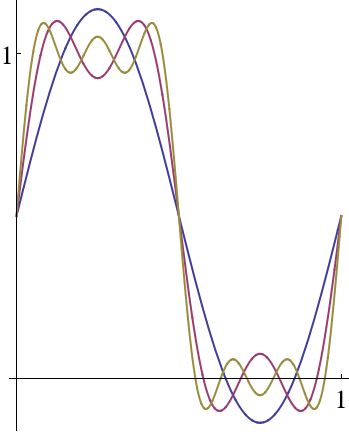## Section10.6Fourier Series Example

Let's consider an example. Suppose $$f(x)$$ describes a square wave of height $$C\text{,}$$ so that

\begin{equation} f(x) = C\, \Theta\left(\frac{L}{2}-x\right)= \begin{cases} C \amp (0\le x\lt \frac{L}{2}) \\ 0 \amp (\frac{L}{2}\lt x\le L) \end{cases}\tag{10.6.1} \end{equation}

where the step function $$\Theta$$ is defined in Section 11.1 (The value of $$f$$ at the single point $$x=L/2$$ doesn't matter).

According to the previous sections, we have

\begin{equation} f(x) = \frac12 a_0 + \sum_{n=1}^\infty a_n \cos\left(\frac{2\pi n x}{L}\right) + \sum_{n=1}^\infty b_n \sin\left(\frac{2\pi n x}{L}\right)\tag{10.6.2} \end{equation}

where

\begin{align} a_0 \amp = \frac{2}{L} \int_0^{\frac{L}{2}}\, C\,dx = C,\tag{10.6.3}\\ a_n \amp = \frac{2}{L} \int_0^{\frac{L}{2}} \cos\left(\frac{2\pi nx}{L}\right) \, C\,dx = 0 ,\tag{10.6.4}\\ b_n \amp = \frac{2}{L} \int_0^{\frac{L}{2}} \sin\left(\frac{2\pi nx}{L}\right) \, C\,dx = \begin{cases} \frac{2C}{\pi n} \amp \hbox{($n$ odd)}\\ 0 \amp \hbox{($n$ even)} \end{cases}\tag{10.6.5} \end{align}

Putting this all together,

\begin{equation} f(x) = C\left(1 + \sum_{\substack{n=1\\ n\hspace{1.5pt}\mathrm{odd}} }^\infty \frac{2}{\pi n} \sin\left(\frac{2\pi nx}{L}\right)\right)\text{.}\tag{10.6.6} \end{equation}

It is instructive to plot the first few terms of this Fourier series and watch the approximation improve as more terms are included, as shown in Figure 10.6.1.Figure 10.6.1. The first few partial sums in the Fourier series for a step function.